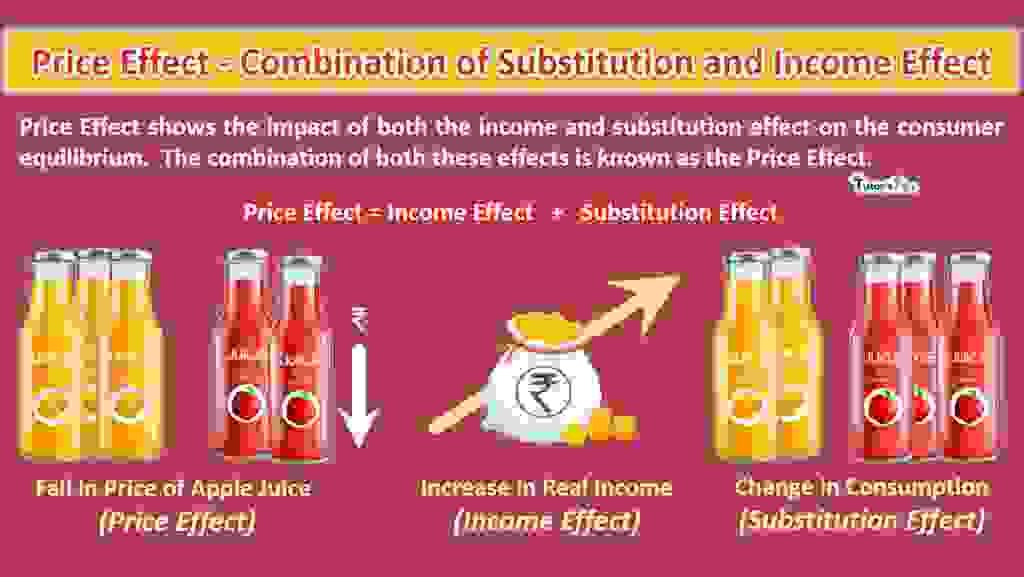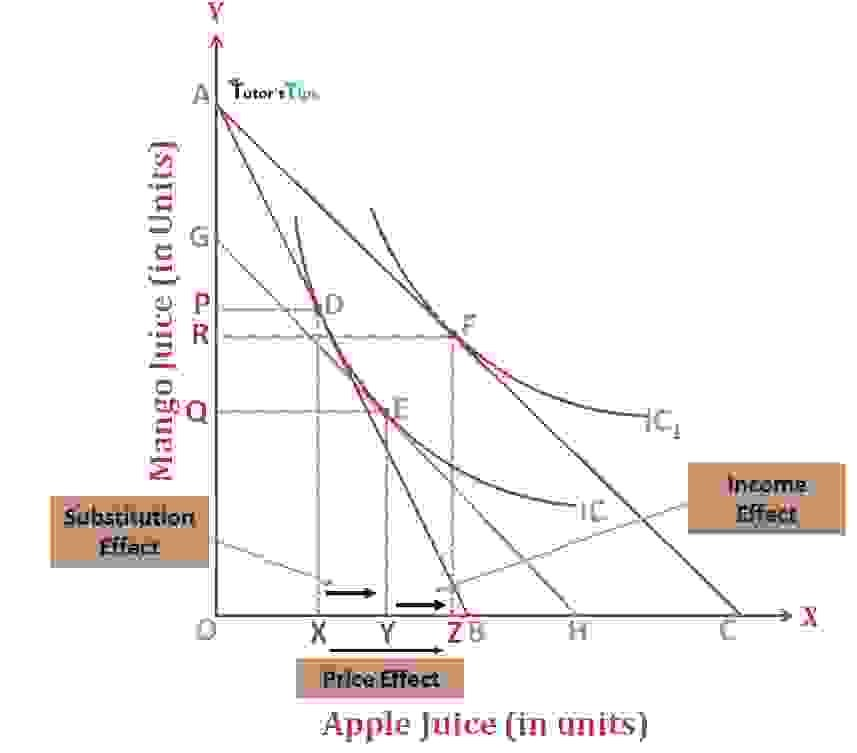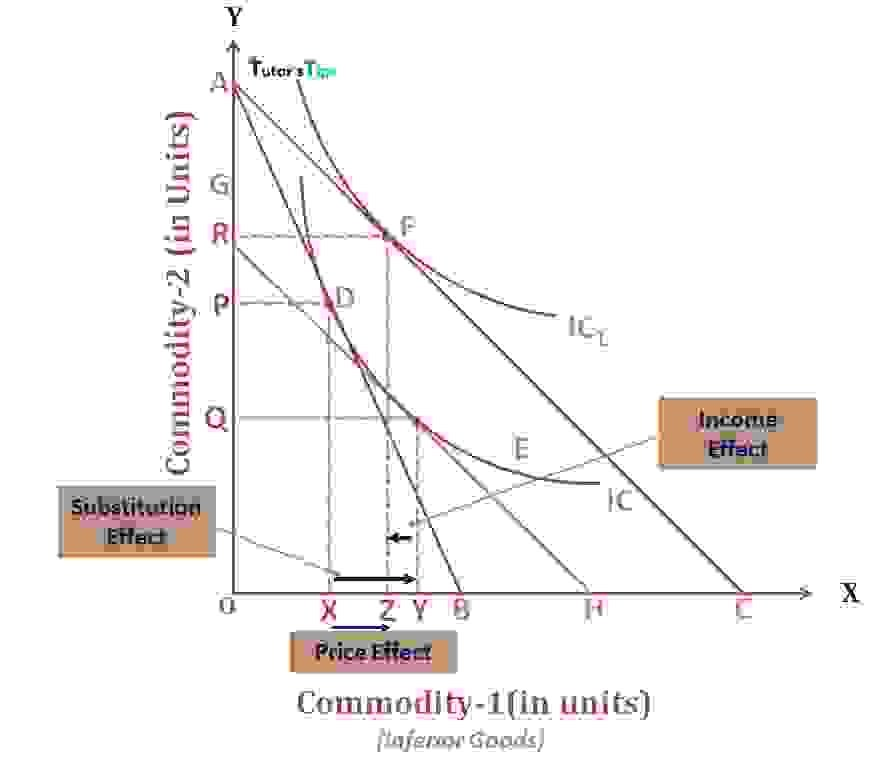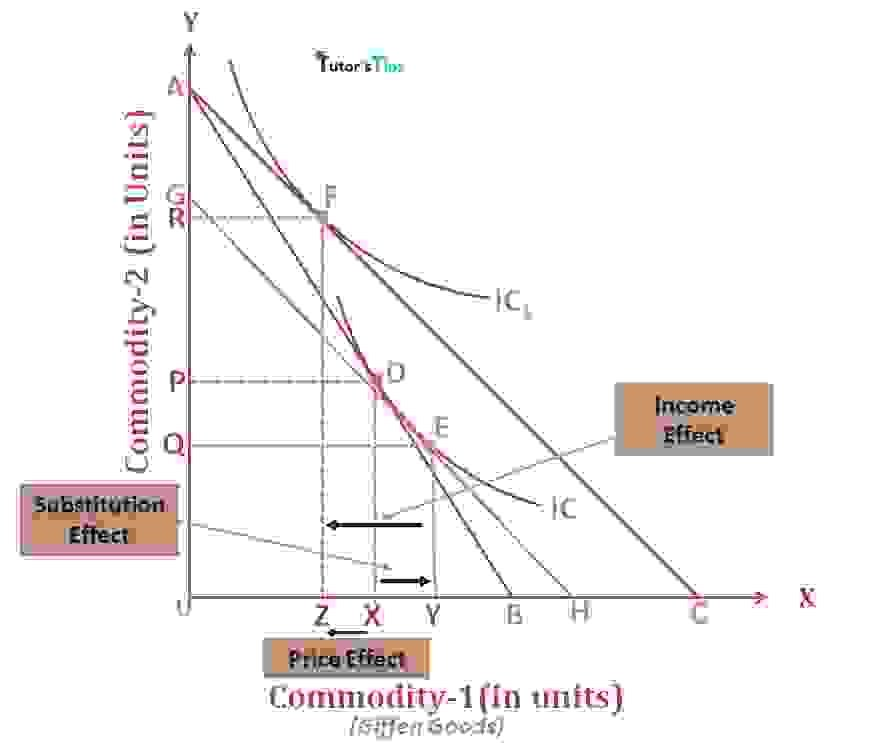# Price Effect – Combination of Substitution and Income EffectThe price Effect shows the impact of both the income and substitution effect on the consumer equilibrium.  The combination of both these effects is known as the Price Effect.

## What is the Price Effect?

It refers to the change in the consumption of the commodities when the price of one of the commodity changes, provided the price of other commodities and income of consumers being the same.

In the words of Lipsey,

“The price effect shows how much satisfaction of the consumer varies due to the change in the consumption of two goods as the price of one changes the price of the other and the money income remains the constant.”

## Price Effect – Combination of Substitution and Income Effect:

When the price of commodity changes, it has two effects :

1. There is a change in the real income of the consumer, leading to a change in the consumption of commodities. It is known as the Income Effect.
2. The change in price results in the substitution of a relatively cheaper commodity for the relatively dearer one. It is known as the Substitution Effect.

The combination of these two effects is called the Price Effect. This combination represents the price-demand relationship in a better way. Price Effect as a combination explains the nature of the response in quantity purchased due to change in price.

Hence,

Price Effect = Substitute Effect + Income Effect

In most cases, the substitute effect and income effect move in the same direction. But, there are also cases, where these both go in opposite directions. A fall in the relative price of one commodity leads to an increase in the consumption of that commodity. Thus, the substitution effect refers to the substitution of a cheaper commodity for the dearer one. It influences the consumer’s purchasing behavior by inducing him to buy more of the cheaper commodity. Hence, the direction of the movement of the substitution effect is certain i.e. negative. But, in the case of Income, it is not certain. It varies with the nature of the commodity.

In short, the price effect comprises of income effect and substitution effect and the direction in which quantity demanded change due to change in the direction of income and substitution effect. The net effect of the price depends upon both these effects. This effect can be explained in three cases:

1. Price Effect for Normal Goods
2. In the case of Inferior Goods and
3. For Giffen Goods

### Price Effect for Normal Goods:

For normal goods, the consumer tends to buy more of a commodity with an increase in income. It implies that the income and quantity demanded are positively related to each other. Whereas the substitute effect and price are negatively related in this case. As the price of a commodity falls, the consumer’s real income increases, which leads to a rise in demand for that commodity. But, for normal goods, Both these effects operate in the same direction.

#### Graphical Representation- Normal Goods:Price Effect for normal goods

In fig, The X-axis shows the quantity of apple juice and the Y-axis shows the quantity of mango juice. AB is the original budget line and the consumer is in equilibrium at point D with indifference curve  IC.

##### Substitution effect:

When the price of apple juice falls, assuming the real income and price of mango juice constant, the budget line shifts to GH. Here, the consumer will move from the equilibrium point D to the new equilibrium point E on the original indifference curve IC. It implies that the consumer is preferring the cheaper apple juice over the mango juice. Thus, the movement of equilibrium points from D to E reflects the substitution effect.

##### Income effect:

If with the fall in the price of apple juice, keeping the price of mango juice unchanged, and there is no reduction in the real income of the consumer. The consumer is able to buy more quantity of apple juice. Thus, the budget line and equilibrium point shifts to AC and point F on a higher indifference curve IC1.  Point F depicts that the consumer is now purchasing more quantities than before. This movement of equilibrium points from E to F reflects the income effect.

##### Price effect:

Thus, in the case of normal goods, the positive income effect and negative substitution effect vary in the same direction, leads to an increase in the quantity demanded of apple juice. It refers to the movement of equilibrium point from D to F. Here, the substitution effect increases the quantity from OX to OY whereas the income effect increases the quantity from OY to OZ.

As a result, the total effect from a fall in the price of apple juice indicated from budget lines AB to AC, the quantity demanded of apple juice increased by XZ. The movement of equilibrium point from D to F represents the increase in quantity demanded of apple juice from OX to OZ units. This total rise in quantity XZ indicates the price effect.

Therefore,

Price Effect = Substitution Effect + Income Effect

XZ= XY+YZ

### In the case of Inferior Goods:

In the case of inferior goods, the consumers tend to buy less of a commodity with a rise in income. It implies, that the income and quantity demanded of inferior goods are inversely related to each other. Here, the substitution effect is also negative. But the negative effect of substitution is more than the negative income effect because a consumer spends a small portion of his income on inferior goods. And when the price of the commodity falls, it affects a very little portion of income. Thus, the negative effect of income generally doesn’t outweigh the substitution effect. As a result, the overall price effect will be negative.

Here, the substitution effect and income effect varies in the opposite direction. When the price of an inferior commodity falls, the substitution effect leads to an increase in the quantity demanded whereas the income effect reduces the quantity demanded. The negative substitution effect outweighs the whole negative income effect. Therefore, the overall effect will be the increase in the quantity demanded of that commodity due to a fall in the price.

#### Graphical Representation- Inferior Goods:Price Effect for inferior goods

In fig, The X-axis shows the quantity of inferior Commodity-1 and the Y-axis shows the quantity of Commodity-2. AB is the original budget line and the consumer is in equilibrium at point D with indifference curve  IC.

##### Substitution effect:

When the price of Commodity-1 falls, keeping the real income and price of Commodity-2 constant, the budget line shifts to GH. Here, the consumer will move from the equilibrium point D to the new equilibrium point at E on the original indifference curve IC. It implies that the consumer is preferring the more inferior commodity-1 with a fall in price. Thus, the movement of equilibrium points from D to E reflects the substitution effect.

##### Income effect:

If with the fall in the price of Commodity-1, keeping the price of Commodity-2 unchanged. Also, there is no reduction in the real income of the consumer. The consumer equilibrium point shifts to F on higher indifference implying the less negative income effect. As we know, a small portion of income is spent on the consumption of inferior goods, the quantity demanded will be reduced from OY to OZ. Thus, the budget line and equilibrium point shifts to AC and point F on a higher indifference curve IC2. This movement of equilibrium points from E to F reflects the income effect.

##### Price effect:

Thus, in the case of inferior goods, the negative income effect and substitution effect varies in the opposite direction, leads to an increase in the quantity demanded of the inferior commodity-1. It refers to the movement of equilibrium point from D to F. Here, the substitution effect increases the quantity from OX to OY whereas the income effect decreases the quantity from OY to OZ.

As a result, the total effect from a fall in the price of the commodity-1 indicated from budget lines AB to AC, the quantity demanded of the inferior commodity increased by XZ. The movement of equilibrium point from D to F represents the increase in quantity demanded of commodity-1 from OX to OZ units. This total rise in quantity XZ indicates the price effect.

Here, Substitution effect (XY) > Negative Income Effect (-YZ)

Therefore,

Price Effect = Substitution Effect + Income Effect

XZ= XY+(-YZ) = XZ

### Price Effect For Giffen Goods:

There are some inferior commodities known as Giffen goods that have a strong negative income effect to outweigh the substitution effect. In the case of Giffen goods, the consumers tend to buy more of a commodity with a rise in income. It implies, that the income and quantity demanded of Giffen goods are inversely related to each other. Here, the substitution effect is also negative. But the negative effect of substitution is less than the negative income effect because a consumer spends a huge portion of his income on Giffen goods. And when the price of the commodity falls, a very large portion of income is affected. Thus, the negative effect of income generally outweighs the substitution effect. As a result, the overall price effect will be negative.

Here, the substitution effect and income effect varies in the opposite direction. When the price of Giffen commodity falls, the substitution effect leads to an increase in the quantity demanded whereas the income effect reduces the quantity demanded. The negative income effect outweighs the whole negative substitution effect. Therefore, the overall effect will be the decrease in the quantity demanded of that commodity due to a fall in the price.

#### Graphical Representation- Giffen Goods:Price Effect for Giffen Goods

In fig, The X-axis shows the quantity of Giffen Commodity-1 and the Y-axis shows the quantity of Commodity-2. AB is the original budget line and the consumer is in equilibrium at point D with indifference curve  IC.

##### Substitution effect:

When the price of Commodity-1 falls, keeping the real income and price of Commodity-2 constant, the budget line shifts to GH. Here, the consumer will move from the equilibrium point D to the new equilibrium point at E on the original indifference curve IC. It implies that the consumer is preferring the more inferior commodity-1 with a fall in price. Thus, the movement of equilibrium points from D to E reflects the substitution effect.

##### Income effect:

If with the fall in the price of Commodity-1, keeping the price of Commodity-2 unchanged, and there is no reduction in the real income of the consumer. The consumer equilibrium point shifts to F on higher indifference implying the less negative income effect. As we know, a huge portion of income is spent on the consumption of inferior goods, the quantity demanded will be reduced from OY to OZ. Thus, the budget line and equilibrium point shifts to AC and point F on a higher indifference curve IC2. This movement of equilibrium points from E to F reflects the income effect.

##### Price effect:

Thus, in the case of Giffen goods, the negative income effect and substitution effect varies in the opposite direction, leads to a reduction in the quantity demanded of the Giffen commodity-1. It refers to the movement of equilibrium point from D to F. Here, the substitution effect increases the quantity from OX to OY whereas the income effect decreases the quantity from OY to OZ.

As a result, the total effect from a fall in the price of the commodity-1 indicated from budget lines AB to AC, the quantity demanded of the Giffen commodity decreased by XZ. The movement of equilibrium point from D to F represents the increase in quantity demanded of commodity-1 from OX to OZ units. This total fall in quantity XZ indicates the price effect.

Here, Substitution effect (XY) < Negative Income Effect (-YZ)

Therefore,

Price Effect = Substitution Effect + Income Effect

XZ= XY+(-)YZ = (-)XZ

Thus, the price,  income, and substitute effect with respect to different commodities can be explained as:

1. Normal Goods: The change in price has a positive income effect and it reinforces the substitution effect and both move in the same direction. Thus, The overall price effect is negative with respect to price.
2. Inferior Goods: The income effect of price change for inferior goods is negative and also, the substitution effect is negative. But, the negative substitution effect is stronger than the income effect. As a result, the overall price effect will be negative with respect to the change in price.
3. Giffen Goods:  In this case, the negative income effect of price change is more powerful than the negative substitution effect. Hence, the overall price effect is positive.

Thanks, and Please share with your friends

Comment if you have any questions.

References:

Introductory Microeconomics – Class 11 – CBSE (2020-21)